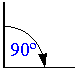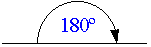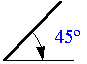# Paper Clip Example

To illustrate the principles behind accelerated testing, consider the following simple example which involves a paper clip. The objective is to determine the mean number of cycles-to-failure of the paper clip. Assume that the use cycle is a 45º bend. The acceleration stress is determined to be the angle to which the paper clip is bent. In this case, assume that the two accelerated bend stresses are 90º and 180º. For the 90º bend, test the paper clip using the following procedure. Use a similar procedure for the 180º and 45º tests.

Note that the paper clips used in this example are Jumbo paper clips that are capable of repeated bending. Different paper clips will yield different results. Additionally, and so that no other stresses are imposed, take caution to assure that the rate at which the paper clips are cycled remains the same across the experiment.

Paper Clip Test

1) Open the Paper Clip.

a. With one hand, hold the clip by the longer, outer loop.
b. With the thumb and forefinger of the other hand, grasp the smaller, inner loop.
c. Pull the smaller, inner loop out and down 90º so that a right angle is formed as shown.

2) Close the Paper Clip.

a. With one hand, continue to hold the clip by the longer, outer loop.
b. With the thumb and forefinger of the other hand, grasp the smaller, inner loop.
c. Push the smaller inner loop up and in 90º so that the smaller loop is returned to the original upright position in line with the larger, outer loop as shown.

This completes one cycle.

3) Repeat steps 1 and 2 until the paper clip breaks. Count and record the cycles-to-failure for each clip.

Analysis

In this experiment, a sample of six paper clips were tested to failure at both 90º and 180º bends. A base test sample of six paper clips was tested at a 45º bend (the assumed use stress level) to confirm the analysis. The cycles-to-failure are given next.

Cycles-to-Failure at 90º16, 17, 18, 21, 22, 23 cycles.

Cycles-to-Failure at 180º4, 4, 5, 6, 6, 8 cycles.

Cycles-to-Failure at 45º58, 63, 65, 72, 78, 86 cycles.

Enter the data in an ALTA standard folio, and analyze the results assuming a lognormal life distribution (fatigue) and an inverse power law relationship (non-thermal) for the life-stress model. The following figures show the analysis and some of the results.

The MTTF at 45º is estimated to be 71.375467 cycles, as shown next.

The next two figures show the analysis of the base data in Weibull++ and the base MTTF estimate. In this case, our accelerated test correctly predicted the MTTF as verified by our base test.